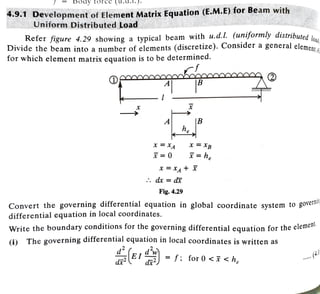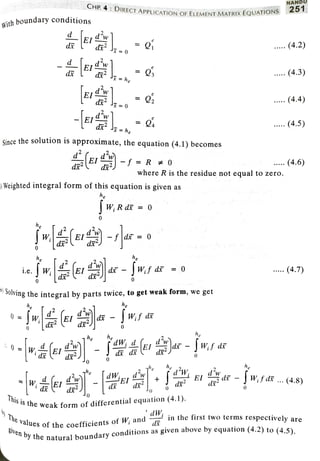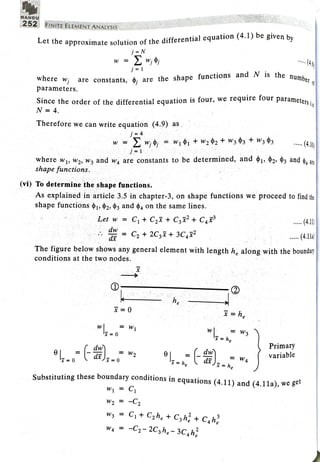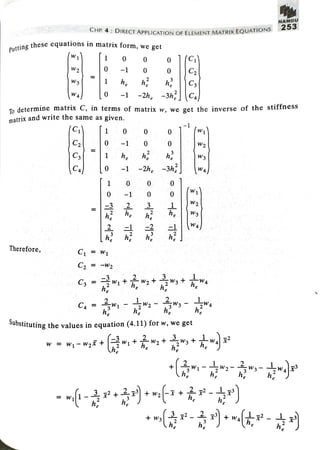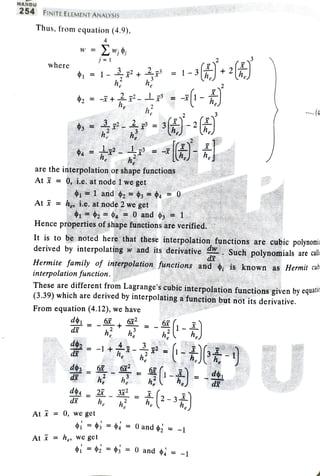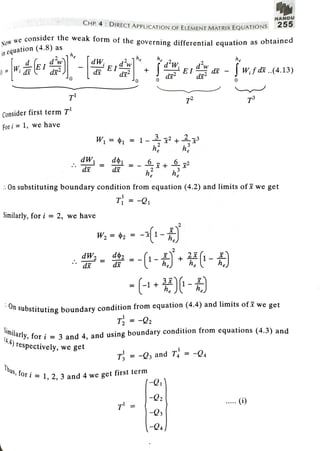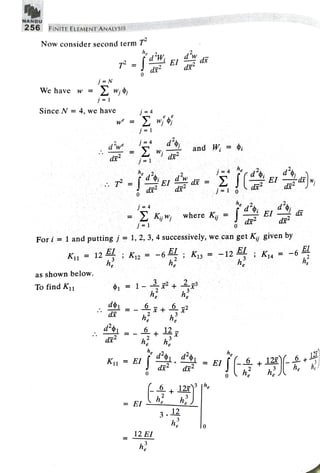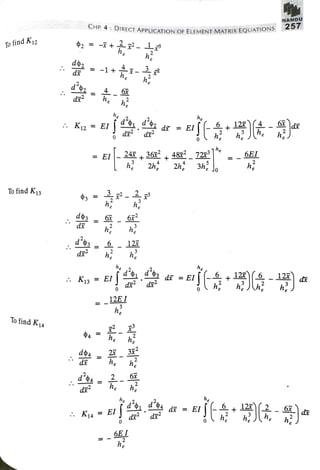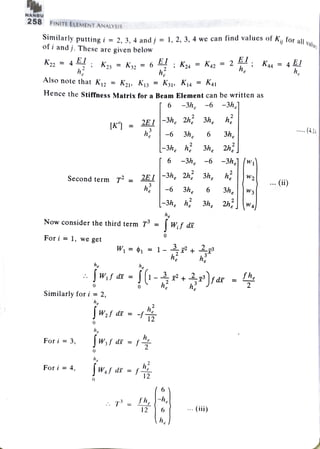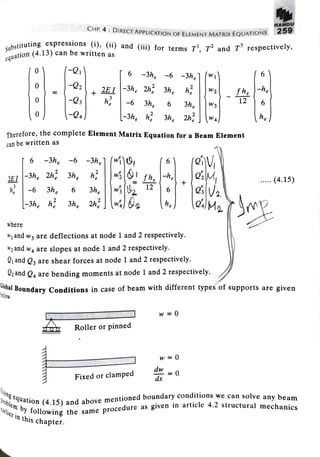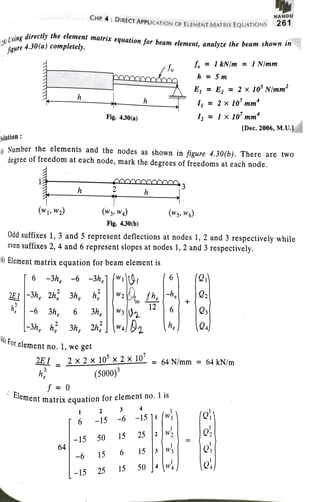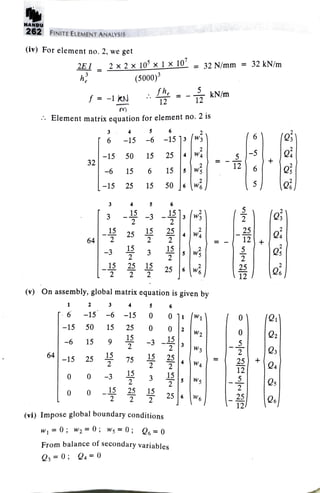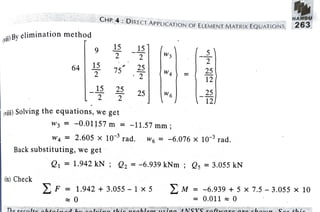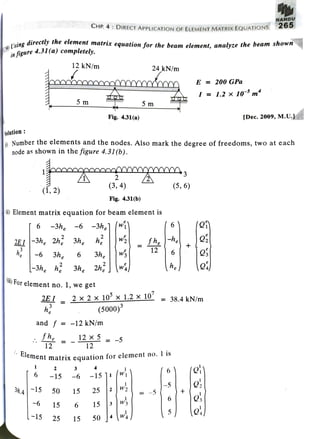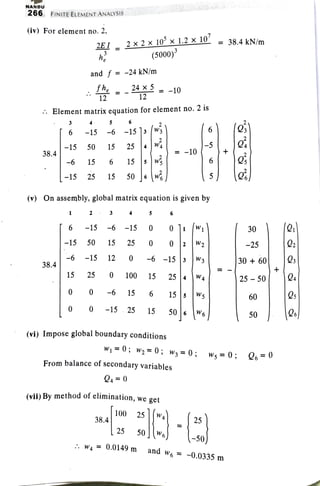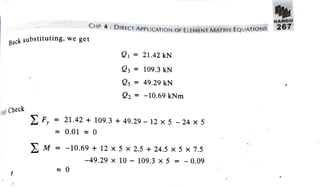1 of 16

### Beam.pdf

1. bOay IOr (u.d.l,). bevelopment of Element Matrix Equation(E.M.E)for Beam with Uniform Distributed Load ************** w. w*VNNAWN S * A**N************ istribui loa DIvide the beam into a number of elements (discretize). Consider a general elemens for which element matrix equation is to be determined. Refer figure 4.29 showing a typical beam with u.d.l. (uniformly distributed ral element .. AE B - B h x XB he x XA = 0 x =XA + dx =d Fig. 4.29 Convert the governing differential equation in global coordinate system to gove differential equation in local coordinates. Write the boundary conditions for the governing differential equation for the eleme ent. ) The governing differential equation in local coordinates is written as (i) EI = f: for 0<F < h, (4 d 2
2. NAN CHP 4 NECTAPPLICATION OF ELEMENTMATRIXEQUATIONS with boundary conditions . (4.2) d2 (4.3) he Er d =0 .. (4.4)) - (4.5) Since the solution is approximate, the equation (4.1) becomes E- -R (4.6) 0 where R is the residue not equal to zero. Weighted integral form of this equation is given as he W R dr = 0 e f|dx = 0 he he 4.7) 0 0 OIng the integral by parts twice, to get weak form, we get he Wif dr he he ne Wif ds d d 0 2 e e - 2 dW -fw.sar4) dr dx n e weak form ofdifferential equation (4.1). This is theN dlues of the coefficients ofW; and n he tirst two terms respectivelv ar. The given by o the natural boundary conditions as given above by equation (4.2) to (4 5).
3. AND 252 FINITE ELEMENT ANALYSIS C approximate solution ofthe differential equation (4.1) be given bu N 49 w = w;¢ j=1 the number where W are constants, o; are the shape functions and N is the parameters. Since the order of the differential equation is four, we require tour parametere: N 4. Therefore we can write equation (4.9) as 4.10 W =1 where w, W2, W3 and w are constants to be determined, and oi, 92, ®3 and o, ae shapefunctions. (vi) To determine the shape functions. As explainedin article 3.5 in chapter-3, on shape functions we proceed to find the shape functions , 2 9s and , on the same lines. Let w = C+ C2 + Cg?+CF° (4.11) dw C2 +2C3 +3C, (4.11a) d The figure below shows any general element with length h, along with the boundary conditions at the two nodes. he I = he W1 W3 Primary dw ),. W2 variable W4 0 Substituting these boundary conditions in equations (4.11) and (4.11a), we ger W C W2 -C2 wsC+ Cah, + C3h +CAh2 = -C2-2C3h-3C,h W4
4. NANDU 253 CHP 4: DIRECT APPLICATION OFELEMENTMATRIXEQUATIONS Putting these equations in matrix form, we get Wi 0 Ca W2 0 -1 0 0 W3 he -1 -2he-3h To determine matrix C, in terms of matrix w, we get the inverse of the stiftneSS matrix and write the same as given. (C 1 0 o1 0 -1 0 0 W2 h h C he W3 c WA 0 -1 -2h 3h 0 W1 -1 0 W2 W3 = Therefore, C1 W1 C2 -wV2 C + + e ne Ca ubstituting the values in equation (4.11) for w, we get w W1-W2X 1 +
5. NANDU 254 FINITE ELEMENT ANALYSIS Thus, from equation (4.9), W= j=1 where -1-+21-3 he TFL |1- h (4 - - are the interpolation or shape functions At = 0,i.e atnode1 weget * * 1 and 2343 Ati = h i.e. at node2 we get 19240 and 3 1 Hence properties ofshapefunctions are verified. 3 It is to be noted here that theseinterpolation functions are cubic polynomia derived by interpolating w and its derivative Such polynomials are calla Hermite family of interpolation functions and o is known as Hermit cub interpolationfunction. These are different from Lagrange'scubicinterpolation functions given byequa (3.39) which are derived by interpolatingafunction butnotits derivative. From equation (4.12), we have de d d do - 1 do h des 2- d h h2 At i = 0, we get 1 = 4 = 0 and ¢2 = -1 At = he, we get = 2 ds = 0 and di = -1
6. NANDU 255 CHP 4: DIRECT APPLICATION OF ELEMENTMATRIXEQUATON we consider the weak form of the governing differential equation as ODLa NOW we in equation (4.8) as e + EI d - JWf d..(4.13) d 0 0 T T2 T Consider first term T' Fori 1, we have W1 = 1 = 1- + e dW - d On substituting boundary condition from equation (4.2) and limits ofX we get T=-1 Similarly, for i = 2, we have W2 2 -1- dW 92 = -1 1-) d di Substituting boundary condition from equation (4.4) and limits ofF we get On T - Thus,fori = 1, 2,3 and 4 we get first term rly, for i =3 and 4, and using boundary condition from equations (4.3) and Similarly, 4.4) respectively, we get T -Q and T = -2 (-e -O (i) T -4
7. NANDU 256 FINITEELEMENTANALYSIS Now consider second term 7 dr d2 0 N We have w =2 wjj j=1 Since N =4, we have d' Wd d w and W i d2 j=? 4 d Ky i =1 where K dp2 d For i = 1 and putting j = 1, 2, 3, 4 successively, we can get K; given by K1 12 K2 -6 K13 =-12 K14 = -6 he h as shown below. To find K1 1 = 1 - h h he K11 E d d2 d2 0 4 12z | EI 3. h 0 = 12EI h
8. NANDU DIRECT APPLICATION OF ELEMENT MATRIXEQUATIONS 1 2-T+ - h To find K12 do 1+ dr e - d2 e d'od d = El K12 EI d d 6EI EI-2424367 , 487 72 3h 2h 2h To find K13 6 d he h2 K13 EI d d 0 0 12EI h To find K14 4 37 d d'42- he K14 .EI d = E - 0 0 6EI
9. NANDU 258 FINITE ELEMENT ANALYSIS Similarly putting i = 2, 3, 4 and j = 1, 2, 3, 4 we can find values of K, for all . of i andj. These are given below value K22=4 he K23 K2 = 6 K2 = Ka2 = 2 Kqa = 4 h EI h AIso note that Ki2 = K21, Kis = K3i» K1a Ka Hence the Stiffness Matrix for a Beam Element can be writter -3h -6 -3h 6 K = 2EI-3he 2h 3h, h 3h (4.14 h -6 3he 6 -3h h 3he 2h 6-3h, -6 -3h1w Second term T = 2EI-3h, 2h2 3h, h W2 h -6 3he 6 3h| 3h, h 3he 2hwa 3he (ii) 3he W3 e Now consider the third term T° = Wf dr Fori= 1, we get W = d1 = 1- - he e WfdR 0 0 Similarly for i = 2, he W2f dz = he Fori= 3, w,f dr For i = 4, WAf dx = f 6 -h T=Ih, 6 12 (ii)
10. NAMDU CHP 4 DIRECTAPPLICATION OF ELEMENT MATRIX EQUATIONS S u b s t i . e q u a t i o n stituting expressions (1), (ii) and (ii) for terms T', T? and T\$ respectively, equation (4.13) can be written as (- 6-3h. -6-3h1 6 2EI-3h 2h 3h h| h W2 h h 0 -Q3 -6 3h 12 6 3h W3 6 - 0 -3h, h 3h 2h2 (he Therefore, the complete Element Matrix Equation for a Beam Element can be written as 6-3h -6 -3h1 (wi| -3h, 2h 3h h|w fh, oM . (4.15) 12 -6 3he 6 3h wg -3h, h 3he 2h w he) where w and wa are deflections at node 1 and 2 respectively. andw are slopes at node 1 and 2 respectively. and Q3 are shear forces at node 1 and 2 respectively. &2 and Q4 are bending moments at node 1 and 2 respectively. tobal Boundary Conditions in case of beam with different types of supports are given elow W 0 Roller or pinned W = 0 dw = 0 Fixed or clamped dx toquation (4.15) and above mentioned boundary conditions we can solve any beam lie following the same procedure as given in article 4.2 structural mechanics ter in this chapter. Oblem by following
11. NANDU 261 CHP 4:DIRECTAPPLICATION OF ELEMENT MATRIX EQUATIONS ) Using directly sing directiy the element matrix equation for beam element, analyze the beam shown in fieure 4.30(a) completely. S, = 1 kN/m = 1 N/mm fo h = 5 m E = E2= 2 x 10 N/mm = 2 x 10 mm h h mmgim7 Fig. 4.30(a) I2= 1 x 10'mm [Dec. 2006, M.U.] Solution: Number the elements and the nodes as shown in figure 4.30(b). There are two degree of freedom at each node, mark the degrees of freedoms at each node h h W1, W2) W3. W) (ws, W) Fig. 4.30(b) Odd suffixes 1, 3 and 5 represent deflections at nodes 1, 2 and 3 respectively while even suffixes 2, 4 and 6 represent slopes at nodes 1, 2 and 3 respectively. ) Element matrix equation for beam element is ( 6 6-3h -6 -3h]"| -he afhe 3h h 2E1-3h 2h h-6 12 Os 6 3h 6 3he -3h, h 3h 2h wal82 ii)For element no. 1, we get 2E 2 x 2 x 10°X 2X10 = 64 N/mm = 64 kN/m (5000) lement matrix equation for element no. 1 is f = 0 2 3 6 -15 -6 -151i -15 50 15 25 :w 64 -6 15 6 15 W 25 15 504 -15
12. NANDU 262 FINITE ELEMENT ANALYSIS (iv) For element no. 2, we get 2E12x2 x10°x1 x10 = 32 N/mm = 32 kN/m h 2EI (5000)3 KN/m f= -1kN 12 Element matrix equation for element no. 2 is 4 6 3 -15 -15 13 W 6 -6 5 50 15 25 4 -15 32 -6 15 6 6 15 5 -15 25 15 506 25 4 V4 64 + -3 3 w 25 (v) On assembly, global matrix equation is given by 2 4 5 6 -15 -6 -15 0 0 11 W 0 -15 50 15 25 0 0 W2 9 -3 -6 15 3 W3 64|-15 25 + 75 25 W4 12 0 0 -3 3 Ws 0 0 (vi) Impose global boundary conditions w = 0; W2 0; Ws 0; Q = 0 From balance of secondary variables , =0; 24= 0
13. MAMDU 63 CHP 4 DIRECT APPLICATION OF ELEMENT MATRIX EQUATION ii)By elimination method 1 9 25 75 2 25 12 64 WA 25 W6 (rii) Solving the equations, we get wa -0.01157 m =-11.57 mm; W42.605 x 10 rad. W6-6.076x 10 rad. W4 Back substituting, we get 1 1.942kN ; Q2 -6.939kNm ; Os = 3.055 kN M = -6.939 + 5x 7.5 -3.055x 10 0.011 0 (x) Check 2F 1.942 + 3.055 -1 x 5 0 Tho w0ilto
14. NANDU CHP 4: DIRECT APPLICATION OF ELEMENT MATRIX EQUATIONS 265 1'sing directly the element matrix equation for the beam element, analyze the beam sn infigure 4.31 (a) completely. 12 kN/m 24 kN/m E = 200 GPa wwww.w w.w 0 wwwwwwwwww. I = 12x 10 m 5 m 5 m Fig. 4.31(a) Dec. 2009, M.U.] Solution: Number the elements and the nodes. Also mark the degree of freedoms, two at each node as shown in the figure 4.31 (b). 2 2 (3, 4) (5, 6) (í, 2) Fig. 4.31(b) li) Element matrix equation for beam element is 6 6 -3he -6 -3h1wi 3he 2E1-3h 2h2 w -he e + 6 h-6 3he 6 3he -3h h 2h he 3he For element no. 1, we get 2EI 2x2x10° x 1.2 X10 = 38.4 kN/m he (5000) and f -12 kN/m fh 12x5 = -5 12 12 Element ma atrix equation for element no. 1 is (e 2 4 6 -is 1( 6 -15 -6 38.415 50 15 25 2 -5 -6 15 6 W2 15 W3 L-15 25 15 50 4wa
15. NANDU 266 FINITE ELEMENT ANALYSIS (iv) For element no.2, 2EI2x 2 x10 X1.2X10 =38.4 kN/m (5000) e and f -24 kN/m 24x =-10 12 Element matrix equation for element no. 2 is 3 5 6 6 -15-6 -1513 W3 -15 50 15 25 w = -10 38.4 -6 15 6 15 Ws 5 -15 25 15 50 w (v) On assembly, global matrix equation is given by 5 -15 -6 -15 0 30 6 -25 -15 50 15 25 0 0 2 W2 W3 -15 12 0 6-15 |3 30 +60 -6 38.4 W4 15 25 0 100 15 25 4w 25 50 00-6 15 6 15 5 W5 60 W6 0 0 -15 25 15 50 Ws 50 (vi) Impose global boundary conditions w0; w =0; w =0; ws=0; ,= 0 From balance of secondary variables Q4= 0 (vii) By method of elimination, we get 100 25w 25 38.4 25 50 -50 W4 0.0149m and w = -0.0335 m
16. CHP 4: DIRECT APPLICATiON OF ELEMENT MATRIX EQUAI NAMDU 267 Backsubstituting, we get 1 21.42 kN Q3 109.3kN Qs = 49.29 kN 2 =-10.69kNm it) Check 2F, 21.42 +109.3 +49.29 12 x 5 -24 x 5 = 0.01 0 M = -10.69 + 12 x 5 x 2.5 + 24.5 x 5 x 7.5 -49.29 x 10 109.3 x 5 = -0.09 0